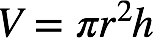# Problem: What is the density of lithium metal in g/cm3 if a cylindrical wire with a diameter of 2.40 mm and a length of 15.0 cm has a mass of 0.3624 g?

###### FREE Expert Solution

Recall: Density is given by:We know the mass of the wire, 0.3624 g. For the volume, we need to calculate the volume of the cylindrical wire. Recall that the volume of a cylinder is given by:where r = radius and h = height or length.

89% (252 ratings)###### Problem Details

What is the density of lithium metal in g/cm3 if a cylindrical wire with a diameter of 2.40 mm and a length of 15.0 cm has a mass of 0.3624 g?